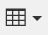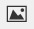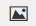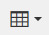PSABM6VZ - Student Facing Task---Acc7.5 Lesson 3 Comparing Proportional Relationships (8.EE.B, 8.EE.B.5)
Part A)

The equationcould represent a variety of different situations.

Write a description of a situation represented by this equation. Decide what quantities x and y represent in your situation.

Part B)

Make a table and a graph that represent the situation.

Create and fill in the table using the table buttonDraw your graph on paper, take a picture, and upload it using the image upload iconIf you do not have the ability to upload an image of your work type "Graph is on paper."

Part A)

Elena and Jada each make money by helping out their neighbors.

Elena babysits. Her earnings are given by the equation y=8.40x, where x represents how many hours she works and y represents how much money she earns.

Jada earns $7 per hour mowing her neighbors' lawns. Who makes more money after working 12 hours? Copied for free from openupresources.org Select one: Part B) How much more do they make? Do not include dollars ($) in your answer.

Type your answer below as a number (example: 5, 3.1, 4 1/2, or 3/2):
Part C)

Explain how you know.

Part D)

What is the rate of change for each situation and what does it mean?

Part E)

How long would it take each person to earn $150? Explain or show your reasoning. Type your answer below: Part A) Clare and Han have summer jobs stuffing envelopes for two different companies. Han earns$15 for every 300 envelopes he finishes.

Clare's earnings can be seen in the table.

 Number of Envelopes Money in Dollars 400 40 900 90

By creating a graph, show how much money each person makes after stuffing 1,500 envelopes.

On a piece of paper, construct your graph. Take a picture and upload it using thebutton. If you cannot, write "Graph is on paper."

Part B)

What is the rate of change for Clare's situation?

Do not include units ($dollars per envelopes) in your answer. Type your answer below as a number (example: 5, 3.1, 4 1/2, or 3/2): Part C) What is the rate of change for Han's situation? Do not include units ($dollars per envelope) in your answer.

Type your answer below as a number (example: 5, 3.1, 4 1/2, or 3/2):
Part D)

What does it mean?

Part E)

Using your graph, determine how much more money one person makes relative to the other after stuffing 1,500 envelopes.

Type your answer below as a number (example: 5, 3.1, 4 1/2, or 3/2):
Part F)

Part A)

Tyler plans to start a lemonade stand and is trying to perfect his recipe for lemonade. He wants to make sure the recipe doesn't use too much lemonade mix (lemon juice and sugar) but still tastes good.

Lemonade Recipe 1 is given by the equation y=4x where x represents the amount of lemonade mix in cups and y represents the amount of cups in water.

Lemonade Recipe 2 is given in the table.

 Lemonade Mix (Cups) Water (Cups) 10 50 13 65 21 105

If Tyler had 16 cups of lemonade mix, how many cups of water would he need for each recipe?

How many cups does he need for Lemonade Recipe 1?

Part B)

How many cups of water does he need for Lemonade Recipe 2?

Type your answer below as a number (example: 5, 3.1, 4 1/2, or 3/2):
Part C)

Explain your reasoning by creating a graph or table.

To create a table, use thebutton.

To create a graph, first create the graph on paper. Then take a picture and upload it using thebutton. If you cannot, write "Graph is on paper."

Part D)

What is the rate of change for each situation?

What is the rate of change for Lemonade Recipe 1?

Type your answer below as a number (example: 5, 3.1, 4 1/2, or 3/2):
Part E)

What is the rate of change for Lemonade Recipe 2?

Type your answer below as a number (example: 5, 3.1, 4 1/2, or 3/2):
Part F)

What does it mean?

Part G)

Tyler has a 5-gallon jug (which holds 80 cups) to use for his lemonade stand and 16 cups of lemonade mix. Which lemonade recipe should he use?

Select one:
Part H)

Part A)

Han and Clare are still stuffing envelopes. Han can stuff 20 envelopes in a minute, and Clare can stuff 10 envelopes in a minute. They start working together on a pile of 1,000 envelopes.

How long does it take them to finish the pile?